# Relighting and Material Editing with Implicit Representations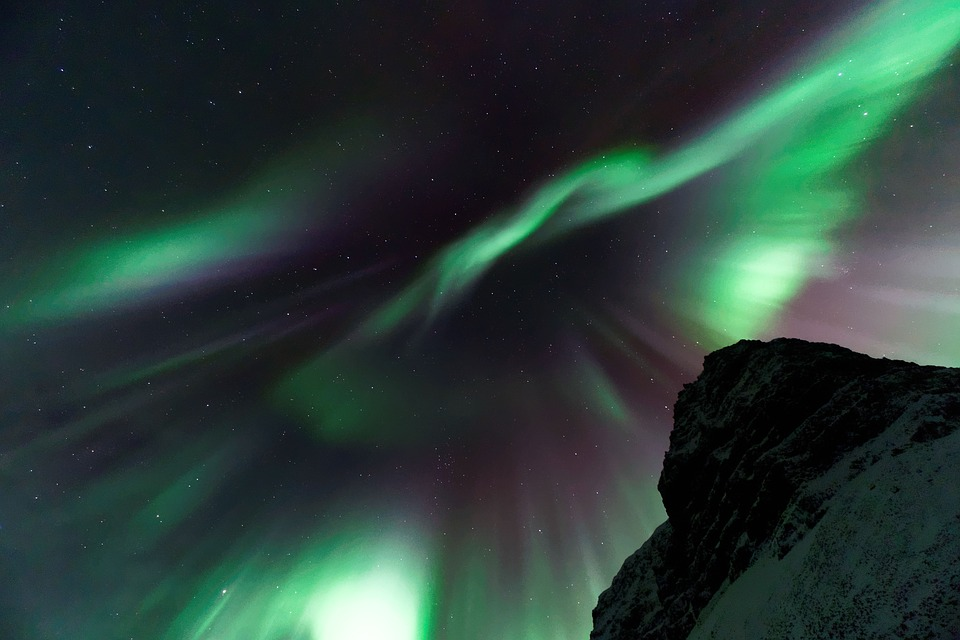Adding relighting and enabling material editing in Implicit Representations

One of the most significant challenges of Computer Vision has been learning the scene from the image. If we can understand the scene and represent it somehow, we will be able to view the scene from new points. This is called Image-Based Rendering (IBR).

The idea is to generate a 3D reconstruction from 2D images and generate unique views. Further, if we wish to retrieve the material and lighting of the scene among other properties, it is referred to as Inverse Rendering.

There are many different ways to represent 3D objects. Classical methods include representing it as a mesh, voxel, or point cloud. These have been extensively studied over the years and have their advantages and disadvantages. Typically they are memory intensive and cannot represent highly detailed objects/scenes or require much computation. While Point clouds can scale well, they usually falter with defining surfaces.We discuss a new class of representations called implicit representations, which are making a lot of noise for all the right reasons. One of them is called the very famous Neural Radiance Fields or NeRF, which has produced over 15-20 variants within the last year itself. NeRF is fantastic at representing an entire scene and view it from any point. However, we cannot edit the scene in any fashion. So we further go through the variants which can perform relighting and material editing while using NeRF as the scene representation.

# Implicit Representation

Recently there has been a new class of representations called implicit representations. The difference mainly is that here we are learning a function that describes the geometry. For example, for a circle, the implicit equation is f(x,y) = x^2+y^2-R^2, where R is the circle’s radius. For any point (x,y), we know if it is on the circle, inside the circle, or outside the circle. Thus, given many points and the information about its position w.r.t circle, we can estimate the circle’s radius.

Similarly, we extend this same idea for 3D as well. We know if points are on, inside, or outside a particular surface, and thus we can estimate our object surface. And what better function approximators are there than neural networks, which are “universal function approximators.”

There are 2 classes of implicit representations depending upon how we want to render the scene. Surface representations aim to find the surface of the object and the corresponding color. In contrast, the volumetric representations do not explicitly look for a surface but instead, try to model the depth of that point and its corresponding color.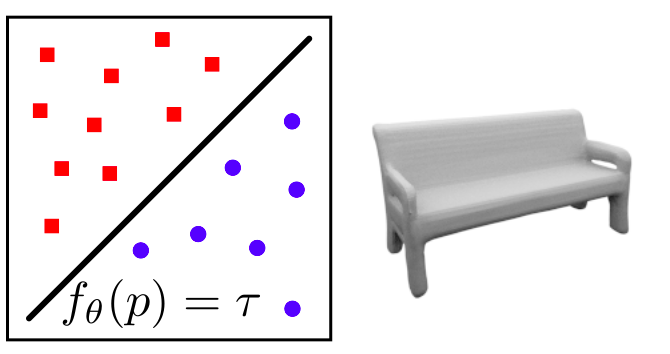Implicit surface representations include Occupancy networks and Signed Distance Fields(SDF). The idea here is that we have a neural network that predicts for a given points its position w.r.t to the object, i.e., if it is on the surface, inside the object, or outside the object. Therefore when we shoot a ray and sample points on it, the network learns their position w.r.t the object. Using this, we can then sample points closer to the surface and find the surface.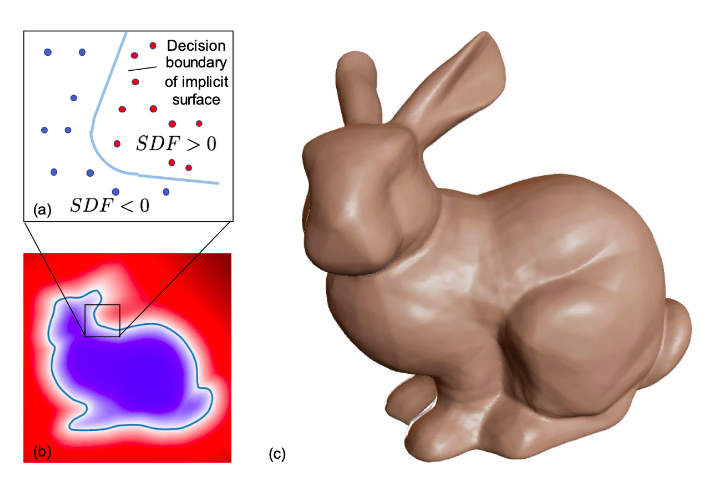The main difference between the occupancy network and the signed distance field is that the occupancy network gives a binary answer. If the point is outside, then it is 0, and if it is inside, it is 1 and on the surface, the value is 0.5. On the other hand, signed distance fields give the distance of the point from the surface. Thus our job would be to find all the points satisfying f(x) = 0. We get positive values inside the object and negative values outside. We can find the surfaces using ray marching or sphere tracing methods. The color of the surface similarly can be found by having a network output for a particular 3D point. While they are pretty famous, they only work where the rays can interact with the surface.

Other works are using implicit surface representations like SRN, Differentiable Volumetric Rendering, PiFU, etc.

# NeRFInstead of finding surfaces of all the objects, we can instead perform volumetric rendering. This is where NeRF and its variants come into the picture. The idea is that instead of learning a surface, we learn the entire volume, which would include not only the objects but also the effects of the medium. Neural Volumes was one of the first works to encode the scene but encoded in a voxel-based representation which is not scalable.

NeRF, on the other hand, uses MLPs to encode the scene. For a ray shot from every pixel, we sample points on the ray. Now every point has a 3D location and a corresponding viewing direction. We pass this 5D vector and obtain the corresponding color and volumetric depth. We do this for all the samples on the ray and then composite them together to get a pixel color. NeRF has two networks, one coarse, which samples points uniformly on the ray, and the other finer network, which does everything the same except we use importance sampling. What it means is that we sample more the points which have more depth, i.e., the objects. Taking viewing direction helps to model view-dependent effects such as specular effects, for eg, reflection of shiny surfaces. Compositing this information uses classic volume rendering techniques, which give us the final image.

So far, the methods that we have read can represent the scene’s geometry well and in a reasonably memory-efficient manner. However, as we have noticed, these methods directly learn and predict the color of a specific point of the surface or scene. Thus it directly bakes in the material and lighting effects, which we cannot edit. Thus although these networks can perform view synthesis pretty well, they cannot change the lighting on the scene or the object’s material.

# Rendering Equation

Before we move ahead, let us understand how computer graphics model material and lighting. Consider a scene with one light source, some objects, and a camera. Now we want to know what a point on the object looks like. We can use some good old physics to compute this. By using energy balance, at a particular point we can say that :I.e. The difference between the power leaving an object, and the power entering it, is equal to the difference between the power it emits and the power it absorbs. In order to enforce energy balance at a surface, exitant radiance Lo must be equal to emitted radiance plus the fraction of incident radiance that is scattered. Emitted radiance is given by, Le and scattered radiance is given by the scattering equation, which givesDo not worry if it looks too technical. At a particular point, we are summing up the contribution of light reflected across a hemisphere. Factor f is called the bidirectional reflectance distribution function or BRDF which tells us how much power will be reflected and absorbed for a particular material. BRDF tells us the properties of a material. There are many models of BRDF like Cook-Torrance, Disney, etc. If BRDF is different for every point, like in a texture, we call it Spatially Varying BRDF or SVBRDF.

There is another version called the surface version of the rendering equation, which we will be referring to in the future as well:Here p’ is our surface, and p is the observer surface or camera. p’’ is the surface from where the light ray is coming on p’, A is all of the surfaces. G is the geometric coupling term which stands for: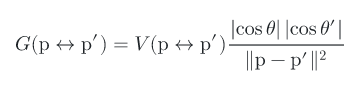V is the visibility function which is one of the surfaces that can see each other else 0.

Now that we understand how material and lighting are modeled, we can understand the various threads of works done to give us material and lighting editing capabilities in implicit representations.

# NeRV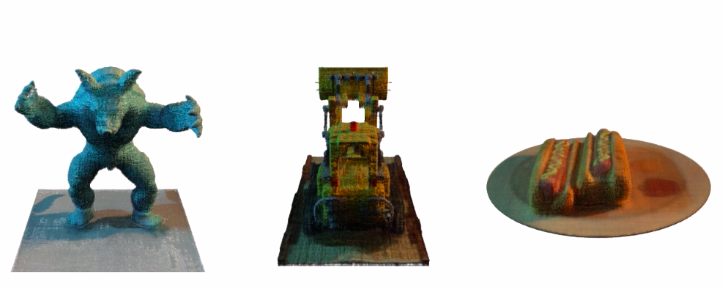Neural Reflectance and Visibility Fields for Relighting and View Synthesis or NeRV attempt to relight the scene with multiple point light sources. In NeRF, we assume that no point sampled on the ray reflects light. However, since we want to perform relighting, we need to model how each point will react to the direct and indirect illumination. Thus instead of each point being an emitter, now we need to compute the reflectance function at each point.So, to begin with, Replace NeRF’s radiance MLP with two MLPs: a “shape” MLP that outputs volume density σ and a “reflectance” MLP that outputs BRDF parameters for any input 3D point. The BRDF model used by the method models it with a 3-dimensional albedo vector and a roughness constant.

Now we can compute the per-point reflectance function analytically for each point along the ray. We would need to query the visibility for each corresponding point the ray hits after hitting one point. However, this operation is very, very expensive, and this is only for direct illumination. For indirect illumination, we need to keep doing the same thing recursively. So instead, what we do is we have a Visibility MLP and Distance MLP. The visibility MLP computes the visibility factor at a given point, whereas the Distance MLP computes the termination point of ray after one bounce.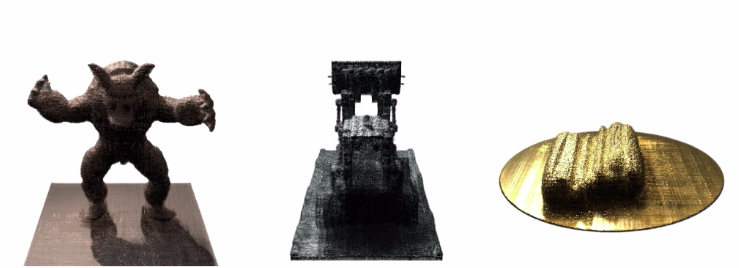So to sum up, here is what happens:

• Sample each ray and query the shape and reflectance MLPs for the volume densities, surface normals, and BRDF parameters
• Shade each point along the ray with direct illumination. Compute this by using the Visibility and BRDF values predicted by corresponding MLP at each sampled point.
• Shade each point along the ray with indirect illumination. Use the predicted endpoint and then compute its effect by sampling along that ray and combining the contribution of each point.
• Combine all these quantities like in NeRF to get the results

NeRV is designed to work with multiple point light sources precisely. Training is compute-intensive. Once trained, we can modify the BRDF parameters and do material editing for the entire scene.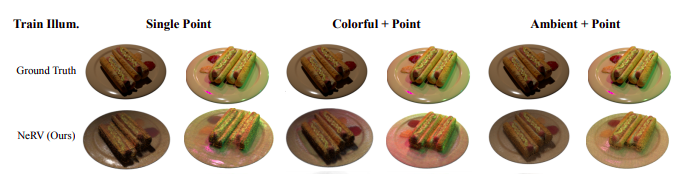TLDR; NeRV uses a Shape MLP to predict volume, a BRDF MLP to predict albedo and roughness, Visibility MLP to predict visibility at each point, and Distance MLP to predict termination of ray after one bounce. The results are combined via the rendering equation for each point and then composited together like NeRF using classical volumetric rendering techniques.

# NeRD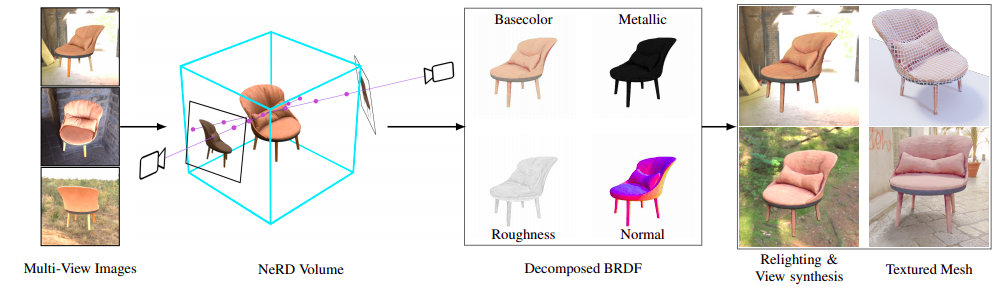Neural Reflectance Decomposition or (NeRD) incorporates Physically-based Rendering or PBR into the NeRF framework. As discussed earlier, the color at a point is the integral over the hemisphere of the product of incoming lighting and SVBRDF. A point could be dark due to material, occlusion, or it is surface normal pointing away. All these considerations are not taken into factor by NeRF as it bakes in the radiance.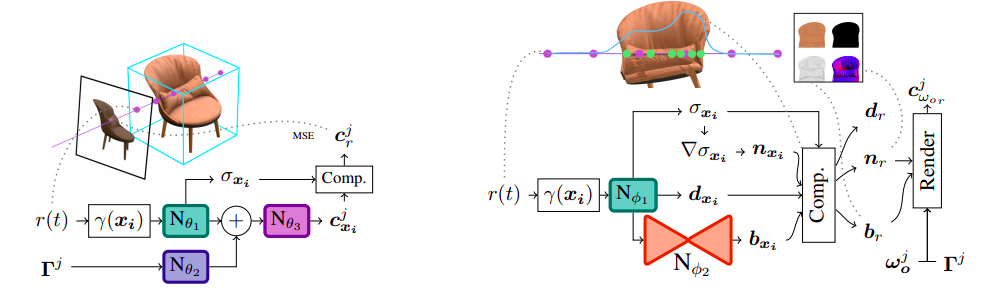NeRD has 2 MLPs, namely the sampling MLP and the decomposition MLP. The sampling MLP outputs a view-independent but illumination-dependent color and the volume density of the scene. Like NeRF, the points are uniformly sampled on the ray in this network, and the volume density is used to importance sample points on the objects in the second network. The final ingredient in the sampling network is the illumination for that particular image. Instead of passing environment light, we pass spherical Gaussian representation of it. Spherical Gaussians are analogous to Fourier Transform in 2D. The reason we do this is that we cannot compute the rendering equation analytically. So instead, we convert it into its Spherical Gaussians form where the integral converts to a product operation. Now we can quickly evaluate the equation. So we are learning illumination, volume, and illumination color from the sampling network.

The decomposition network extends the second network of NeRF. Along with color and volume density, we also compute a vector and pass it through another small autoencoder to output the BRDF parameters of the object. Here the BRDF parameters are different from NeRV as the model outputs albedo, metallic, and roughness. The autoencoder is there to optimize the training and improve results. Finally, we combine the outputs like NeRF and pass them through classical volume rendering to output the image.NeRD makes the color of the scene view independent and learns the lighting and the BRDF properties. Once learned, it is straightforward to model relighting as we know how illumination is combined to give color.

TLDR; NeRD decomposes the scene and learns the illumination and BRDF parameters of the scene separately. The two networks of NeRF are augmented to learn view independent and illumination dependent color, and once trained, it is straightforward to perform relighting.

# NeRFactorNeRFactor is very different from all the works we have seen so far as it distills the trained NeRF model, which no other work in this line has done so far as NeRF works on the entire volume while this works on surface points. It can perform free-viewpoint relighting as well as material editing.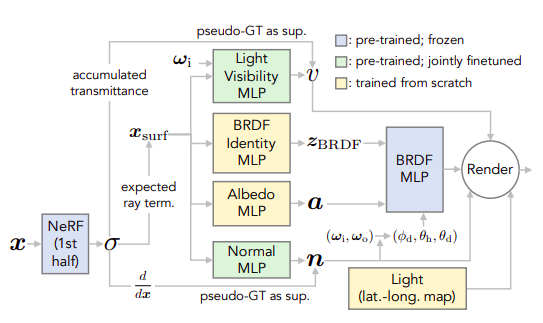First, we train a NeRF network on the scene. We then keep the coarse network and freeze its weights. We then train a BRDF MLP on the MERL dataset. The MERL dataset contains reflectance functions of 100 different materials. Then we initialize a normal map and a visibility map using the predicted volume from the pretrained NeRF. These maps are very noisy, and hence instead of freezing them, we take them as initializations.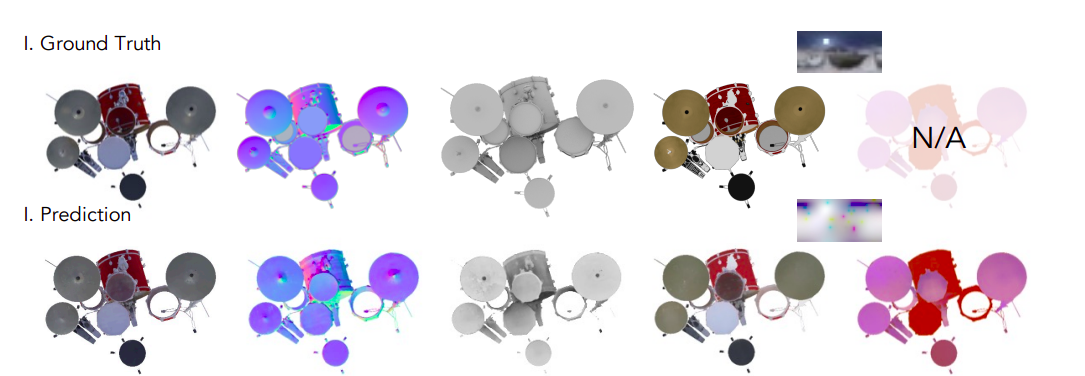Now we first predict where the ray will hit the surface. Using that as an input, we train 4 MLPs, namely Light Visibility MLP, BRDF Identity MLP, Albedo MLP, and Normal MLP. Since we have our initializations for visibility and normals, they are called pretrained. Now we input the surface points and get the outputs. The BRDF MLP outputs a latent vector z which will be used for material editing. The albedo network handles the diffuse color component. We also estimate lighting for each surface point. NeRFactor can separate shadows from albedo by explicitly modeling light visibility and synthesize realistic soft or hard shadows under arbitrary lighting conditions. All the outputs are then combined like in NeRF and rendered using classical volumetric rendering.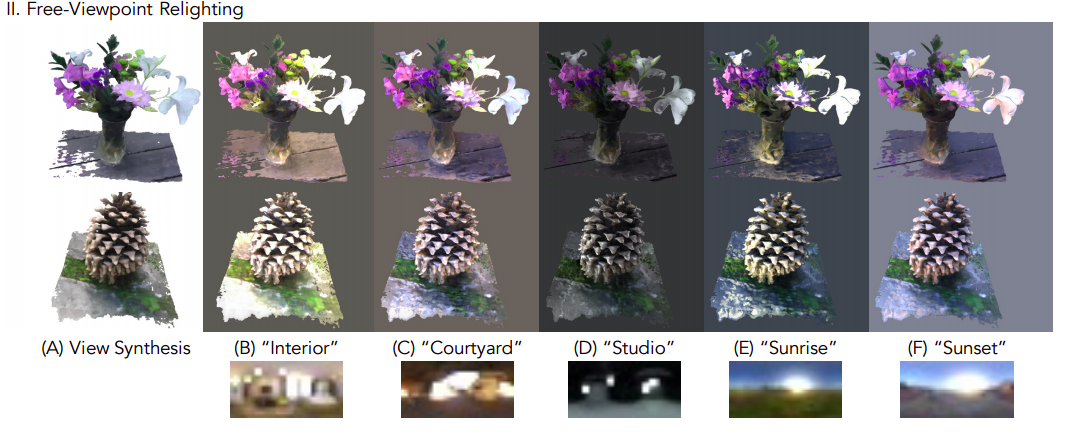NeRFactor does not predict the BRDF parameters. Instead, it learns a latent vector that can be easily used to render material edits. On top of that, it is not taking points anywhere on the volume but only on the object’s surface.

TLDR; NerFactor uses a trained NeRF to initialize normal and visibility maps and a trained BRDF MLP to learn the latent vector representation. It then searches for the points on the surface of the object and learns its various parameters. After learning, we can perform relighting and material editing.

# Takeaways

We go through 3 methods that have empowered NeRF with atleast relighting capabilities. NeRV does this by computing the effects of direct and indirect illumination at each point and approximates visibility and ray termination using MLP. On the other hand, NeRFactor decomposes by first finding the object’s surface and then learns the lighting and BRDF parameters(in this case, a latent vector representation). NeRD is somewhere in the middle where its decomposition network computes the surface normal of the object using weighted sampling and uses it to render the scene but still runs for all points in the volume.

We observe that more and more methods are going towards a surface representation to gain more control over the editing of the scene as we are not very concerned with what happens to the medium. Very excited to see which direction this field takes two more papers down the line.